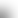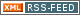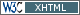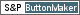www.LinuxHowtos.org howtos, tips&tricks and tutorials for linuxfrom small one page howto to huge articles all in one place

search text in:

Poll
Which linux distribution do you use?

poll results

using iotop to find disk usage hogs

using iotop to find disk usage hogs

words:

887

views:

165658

userrating:

average rating: 1.8 (88 votes) (1=very good 6=terrible)

May 25th. 2007:
Words

486

Views

248876

Workaround and fixes for the current Core Dump Handling vulnerability affected kernels

Workaround and fixes for the current Core Dump Handling vulnerability affected kernels

words:

161

views:

133625

userrating:

average rating: 1.3 (28 votes) (1=very good 6=terrible)

April, 26th. 2006:

You are here: manpages

# des

Section: OpenSSL (3)
Updated: 2017-05-25

## NAME

DES_random_key, DES_set_key, DES_key_sched, DES_set_key_checked, DES_set_key_unchecked, DES_set_odd_parity, DES_is_weak_key, DES_ecb_encrypt, DES_ecb2_encrypt, DES_ecb3_encrypt, DES_ncbc_encrypt, DES_cfb_encrypt, DES_ofb_encrypt, DES_pcbc_encrypt, DES_cfb64_encrypt, DES_ofb64_encrypt, DES_xcbc_encrypt, DES_ede2_cbc_encrypt, DES_ede2_cfb64_encrypt, DES_ede2_ofb64_encrypt, DES_ede3_cbc_encrypt, DES_ede3_cbcm_encrypt, DES_ede3_cfb64_encrypt, DES_ede3_ofb64_encrypt, DES_cbc_cksum, DES_quad_cksum, DES_string_to_key, DES_string_to_2keys, DES_fcrypt, DES_crypt, DES_enc_read, DES_enc_write - DES encryption

## SYNOPSIS

``` #include <openssl/des.h>

void DES_random_key(DES_cblock *ret);

int DES_set_key(const_DES_cblock *key, DES_key_schedule *schedule);
int DES_key_sched(const_DES_cblock *key, DES_key_schedule *schedule);
int DES_set_key_checked(const_DES_cblock *key,
DES_key_schedule *schedule);
void DES_set_key_unchecked(const_DES_cblock *key,
DES_key_schedule *schedule);

void DES_set_odd_parity(DES_cblock *key);
int DES_is_weak_key(const_DES_cblock *key);

void DES_ecb_encrypt(const_DES_cblock *input, DES_cblock *output,
DES_key_schedule *ks, int enc);
void DES_ecb2_encrypt(const_DES_cblock *input, DES_cblock *output,
DES_key_schedule *ks1, DES_key_schedule *ks2, int enc);
void DES_ecb3_encrypt(const_DES_cblock *input, DES_cblock *output,
DES_key_schedule *ks1, DES_key_schedule *ks2,
DES_key_schedule *ks3, int enc);

void DES_ncbc_encrypt(const unsigned char *input, unsigned char *output,
long length, DES_key_schedule *schedule, DES_cblock *ivec,
int enc);
void DES_cfb_encrypt(const unsigned char *in, unsigned char *out,
int numbits, long length, DES_key_schedule *schedule,
DES_cblock *ivec, int enc);
void DES_ofb_encrypt(const unsigned char *in, unsigned char *out,
int numbits, long length, DES_key_schedule *schedule,
DES_cblock *ivec);
void DES_pcbc_encrypt(const unsigned char *input, unsigned char *output,
long length, DES_key_schedule *schedule, DES_cblock *ivec,
int enc);
void DES_cfb64_encrypt(const unsigned char *in, unsigned char *out,
long length, DES_key_schedule *schedule, DES_cblock *ivec,
int *num, int enc);
void DES_ofb64_encrypt(const unsigned char *in, unsigned char *out,
long length, DES_key_schedule *schedule, DES_cblock *ivec,
int *num);

void DES_xcbc_encrypt(const unsigned char *input, unsigned char *output,
long length, DES_key_schedule *schedule, DES_cblock *ivec,
const_DES_cblock *inw, const_DES_cblock *outw, int enc);

void DES_ede2_cbc_encrypt(const unsigned char *input,
unsigned char *output, long length, DES_key_schedule *ks1,
DES_key_schedule *ks2, DES_cblock *ivec, int enc);
void DES_ede2_cfb64_encrypt(const unsigned char *in,
unsigned char *out, long length, DES_key_schedule *ks1,
DES_key_schedule *ks2, DES_cblock *ivec, int *num, int enc);
void DES_ede2_ofb64_encrypt(const unsigned char *in,
unsigned char *out, long length, DES_key_schedule *ks1,
DES_key_schedule *ks2, DES_cblock *ivec, int *num);

void DES_ede3_cbc_encrypt(const unsigned char *input,
unsigned char *output, long length, DES_key_schedule *ks1,
DES_key_schedule *ks2, DES_key_schedule *ks3, DES_cblock *ivec,
int enc);
void DES_ede3_cbcm_encrypt(const unsigned char *in, unsigned char *out,
long length, DES_key_schedule *ks1, DES_key_schedule *ks2,
DES_key_schedule *ks3, DES_cblock *ivec1, DES_cblock *ivec2,
int enc);
void DES_ede3_cfb64_encrypt(const unsigned char *in, unsigned char *out,
long length, DES_key_schedule *ks1, DES_key_schedule *ks2,
DES_key_schedule *ks3, DES_cblock *ivec, int *num, int enc);
void DES_ede3_ofb64_encrypt(const unsigned char *in, unsigned char *out,
long length, DES_key_schedule *ks1,
DES_key_schedule *ks2, DES_key_schedule *ks3,
DES_cblock *ivec, int *num);

DES_LONG DES_cbc_cksum(const unsigned char *input, DES_cblock *output,
long length, DES_key_schedule *schedule,
const_DES_cblock *ivec);
DES_LONG DES_quad_cksum(const unsigned char *input, DES_cblock output[],
long length, int out_count, DES_cblock *seed);
void DES_string_to_key(const char *str, DES_cblock *key);
void DES_string_to_2keys(const char *str, DES_cblock *key1,
DES_cblock *key2);

char *DES_fcrypt(const char *buf, const char *salt, char *ret);
char *DES_crypt(const char *buf, const char *salt);

int DES_enc_read(int fd, void *buf, int len, DES_key_schedule *sched,
DES_cblock *iv);
int DES_enc_write(int fd, const void *buf, int len,
DES_key_schedule *sched, DES_cblock *iv);

```

## DESCRIPTION

This library contains a fast implementation of the DES encryption algorithm.

There are two phases to the use of DES encryption. The first is the generation of a DES_key_schedule from a key, the second is the actual encryption. A DES key is of type DES_cblock. This type is consists of 8 bytes with odd parity. The least significant bit in each byte is the parity bit. The key schedule is an expanded form of the key; it is used to speed the encryption process.

DES_random_key() generates a random key. The PRNG must be seeded prior to using this function (see rand(3)). If the PRNG could not generate a secure key, 0 is returned.

Before a DES key can be used, it must be converted into the architecture dependent DES_key_schedule via the DES_set_key_checked() or DES_set_key_unchecked() function.

DES_set_key_checked() will check that the key passed is of odd parity and is not a weak or semi-weak key. If the parity is wrong, then -1 is returned. If the key is a weak key, then -2 is returned. If an error is returned, the key schedule is not generated.

DES_set_key() works like DES_set_key_checked() if the DES_check_key flag is non-zero, otherwise like DES_set_key_unchecked(). These functions are available for compatibility; it is recommended to use a function that does not depend on a global variable.

DES_set_odd_parity() sets the parity of the passed key to odd.

DES_is_weak_key() returns 1 if the passed key is a weak key, 0 if it is ok.

The following routines mostly operate on an input and output stream of DES_cblocks.

DES_ecb_encrypt() is the basic DES encryption routine that encrypts or decrypts a single 8-byte DES_cblock in electronic code book (ECB) mode. It always transforms the input data, pointed to by input, into the output data, pointed to by the output argument. If the encrypt argument is non-zero (DES_ENCRYPT), the input (cleartext) is encrypted in to the output (ciphertext) using the key_schedule specified by the schedule argument, previously set via DES_set_key. If encrypt is zero (DES_DECRYPT), the input (now ciphertext) is decrypted into the output (now cleartext). Input and output may overlap. DES_ecb_encrypt() does not return a value.

DES_ecb3_encrypt() encrypts/decrypts the input block by using three-key Triple-DES encryption in ECB mode. This involves encrypting the input with ks1, decrypting with the key schedule ks2, and then encrypting with ks3. This routine greatly reduces the chances of brute force breaking of DES and has the advantage of if ks1, ks2 and ks3 are the same, it is equivalent to just encryption using ECB mode and ks1 as the key.

The macro DES_ecb2_encrypt() is provided to perform two-key Triple-DES encryption by using ks1 for the final encryption.

DES_ncbc_encrypt() encrypts/decrypts using the cipher-block-chaining (CBC) mode of DES. If the encrypt argument is non-zero, the routine cipher-block-chain encrypts the cleartext data pointed to by the input argument into the ciphertext pointed to by the output argument, using the key schedule provided by the schedule argument, and initialization vector provided by the ivec argument. If the length argument is not an integral multiple of eight bytes, the last block is copied to a temporary area and zero filled. The output is always an integral multiple of eight bytes.

DES_xcbc_encrypt() is RSA's DESX mode of DES. It uses inw and outw to 'whiten' the encryption. inw and outw are secret (unlike the iv) and are as such, part of the key. So the key is sort of 24 bytes. This is much better than CBC DES.

DES_ede3_cbc_encrypt() implements outer triple CBC DES encryption with three keys. This means that each DES operation inside the CBC mode is an "C=E(ks3,D(ks2,E(ks1,M)))". This mode is used by SSL.

The DES_ede2_cbc_encrypt() macro implements two-key Triple-DES by reusing ks1 for the final encryption. "C=E(ks1,D(ks2,E(ks1,M)))". This form of Triple-DES is used by the RSAREF library.

DES_pcbc_encrypt() encrypt/decrypts using the propagating cipher block chaining mode used by Kerberos v4. Its parameters are the same as DES_ncbc_encrypt().

DES_cfb_encrypt() encrypt/decrypts using cipher feedback mode. This method takes an array of characters as input and outputs and array of characters. It does not require any padding to 8 character groups. Note: the ivec variable is changed and the new changed value needs to be passed to the next call to this function. Since this function runs a complete DES ECB encryption per numbits, this function is only suggested for use when sending small numbers of characters.

DES_cfb64_encrypt() implements CFB mode of DES with 64bit feedback. Why is this useful you ask? Because this routine will allow you to encrypt an arbitrary number of bytes, no 8 byte padding. Each call to this routine will encrypt the input bytes to output and then update ivec and num. num contains 'how far' we are though ivec. If this does not make much sense, read more about cfb mode of DES :-).

DES_ede3_cfb64_encrypt() and DES_ede2_cfb64_encrypt() is the same as DES_cfb64_encrypt() except that Triple-DES is used.

DES_ofb_encrypt() encrypts using output feedback mode. This method takes an array of characters as input and outputs and array of characters. It does not require any padding to 8 character groups. Note: the ivec variable is changed and the new changed value needs to be passed to the next call to this function. Since this function runs a complete DES ECB encryption per numbits, this function is only suggested for use when sending small numbers of characters.

DES_ofb64_encrypt() is the same as DES_cfb64_encrypt() using Output Feed Back mode.

DES_ede3_ofb64_encrypt() and DES_ede2_ofb64_encrypt() is the same as DES_ofb64_encrypt(), using Triple-DES.

The following functions are included in the DES library for compatibility with the MIT Kerberos library.

DES_cbc_cksum() produces an 8 byte checksum based on the input stream (via CBC encryption). The last 4 bytes of the checksum are returned and the complete 8 bytes are placed in output. This function is used by Kerberos v4. Other applications should use EVP_DigestInit(3) etc. instead.

DES_quad_cksum() is a Kerberos v4 function. It returns a 4 byte checksum from the input bytes. The algorithm can be iterated over the input, depending on out_count, 1, 2, 3 or 4 times. If output is non-NULL, the 8 bytes generated by each pass are written into output.

The following are DES-based transformations:

DES_fcrypt() is a fast version of the Unix crypt(3) function. This version takes only a small amount of space relative to other fast crypt() implementations. This is different to the normal crypt in that the third parameter is the buffer that the return value is written into. It needs to be at least 14 bytes long. This function is thread safe, unlike the normal crypt.

DES_crypt() is a faster replacement for the normal system crypt(). This function calls DES_fcrypt() with a static array passed as the third parameter. This emulates the normal non-thread safe semantics of crypt(3).

DES_enc_write() writes len bytes to file descriptor fd from buffer buf. The data is encrypted via pcbc_encrypt (default) using sched for the key and iv as a starting vector. The actual data send down fd consists of 4 bytes (in network byte order) containing the length of the following encrypted data. The encrypted data then follows, padded with random data out to a multiple of 8 bytes.

DES_enc_read() is used to read len bytes from file descriptor fd into buffer buf. The data being read from fd is assumed to have come from DES_enc_write() and is decrypted using sched for the key schedule and iv for the initial vector.

Warning: The data format used by DES_enc_write() and DES_enc_read() has a cryptographic weakness: When asked to write more than MAXWRITE bytes, DES_enc_write() will split the data into several chunks that are all encrypted using the same IV. So don't use these functions unless you are sure you know what you do (in which case you might not want to use them anyway). They cannot handle non-blocking sockets. DES_enc_read() uses an internal state and thus cannot be used on multiple files.

DES_rw_mode is used to specify the encryption mode to use with DES_enc_read() and DES_end_write(). If set to DES_PCBC_MODE (the default), DES_pcbc_encrypt is used. If set to DES_CBC_MODE DES_cbc_encrypt is used.

## NOTES

Single-key DES is insecure due to its short key size. ECB mode is not suitable for most applications; see des_modes(7).

The evp(3) library provides higher-level encryption functions.

## BUGS

DES_3cbc_encrypt() is flawed and must not be used in applications.

DES_cbc_encrypt() does not modify ivec; use DES_ncbc_encrypt() instead.

DES_cfb_encrypt() and DES_ofb_encrypt() operates on input of 8 bits. What this means is that if you set numbits to 12, and length to 2, the first 12 bits will come from the 1st input byte and the low half of the second input byte. The second 12 bits will have the low 8 bits taken from the 3rd input byte and the top 4 bits taken from the 4th input byte. The same holds for output. This function has been implemented this way because most people will be using a multiple of 8 and because once you get into pulling bytes input bytes apart things get ugly!

DES_string_to_key() is available for backward compatibility with the MIT library. New applications should use a cryptographic hash function. The same applies for DES_string_to_2key().

## CONFORMING TO

ANSI X3.106

The des library was written to be source code compatible with the MIT Kerberos library.

crypt(3), des_modes(7), evp(3), rand(3)

## HISTORY

In OpenSSL 0.9.7, all des_ functions were renamed to DES_ to avoid clashes with older versions of libdes. Compatibility des_ functions are provided for a short while, as well as crypt(). Declarations for these are in <openssl/des_old.h>. There is no DES_ variant for des_random_seed(). This will happen to other functions as well if they are deemed redundant (des_random_seed() just calls RAND_seed() and is present for backward compatibility only), buggy or already scheduled for removal.

des_cbc_cksum(), des_cbc_encrypt(), des_ecb_encrypt(), des_is_weak_key(), des_key_sched(), des_pcbc_encrypt(), des_quad_cksum(), des_random_key() and des_string_to_key() are available in the MIT Kerberos library; des_check_key_parity(), des_fixup_key_parity() and des_is_weak_key() are available in newer versions of that library.

des_set_key_checked() and des_set_key_unchecked() were added in OpenSSL 0.9.5.

des_generate_random_block(), des_init_random_number_generator(), des_new_random_key(), des_set_random_generator_seed() and des_set_sequence_number() and des_rand_data() are used in newer versions of Kerberos but are not implemented here.

des_random_key() generated cryptographically weak random data in SSLeay and in OpenSSL prior version 0.9.5, as well as in the original MIT library.

## AUTHOR

Eric Young (eay@cryptsoft.com). Modified for the OpenSSL project (http://www.openssl.org).

## Index

NAME
SYNOPSIS
DESCRIPTION
NOTES
BUGS
CONFORMING TO
HISTORY
AUTHOR||- Powered by- Running on-
Copyright 2004-2020 Sascha Nitsch Unternehmensberatung GmbH::- Level Triple-A Conformance to Web Content Accessibility Guidelines 1.0 -
- Copyright and legal notices -